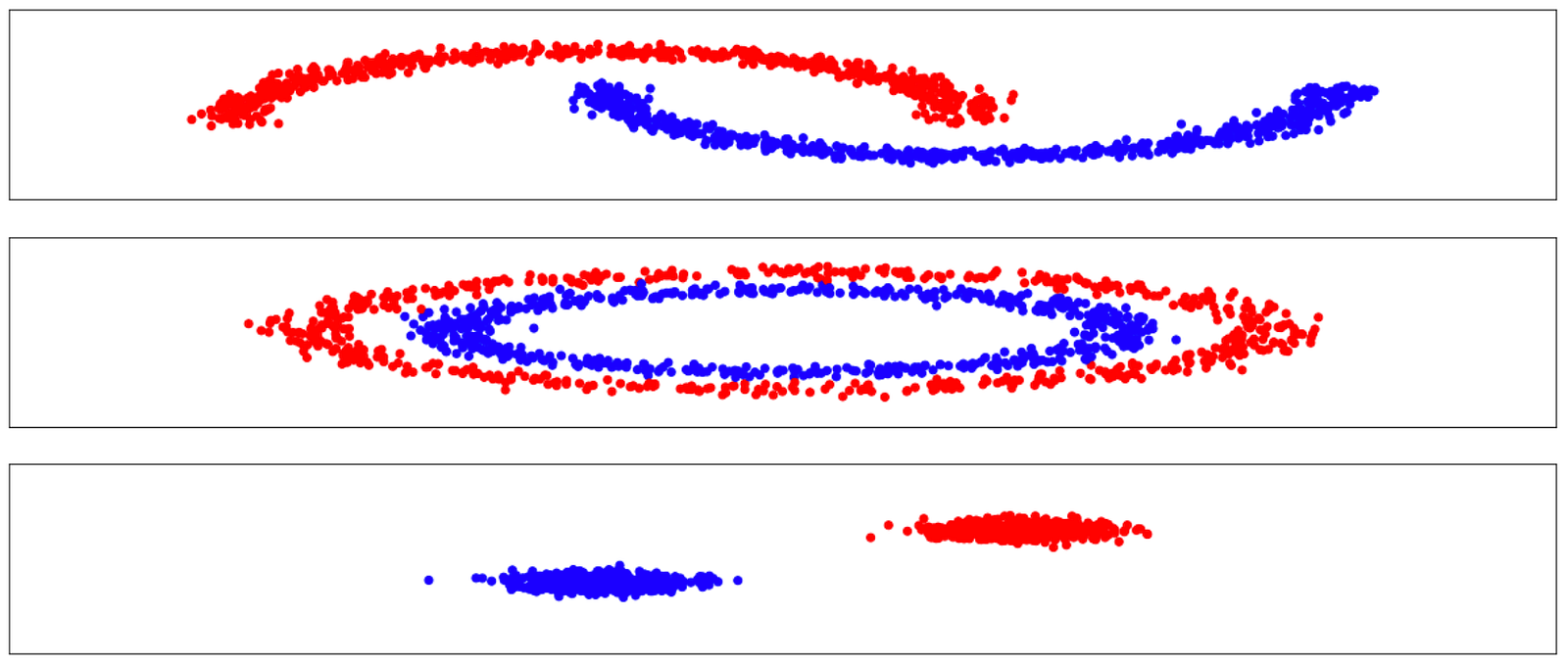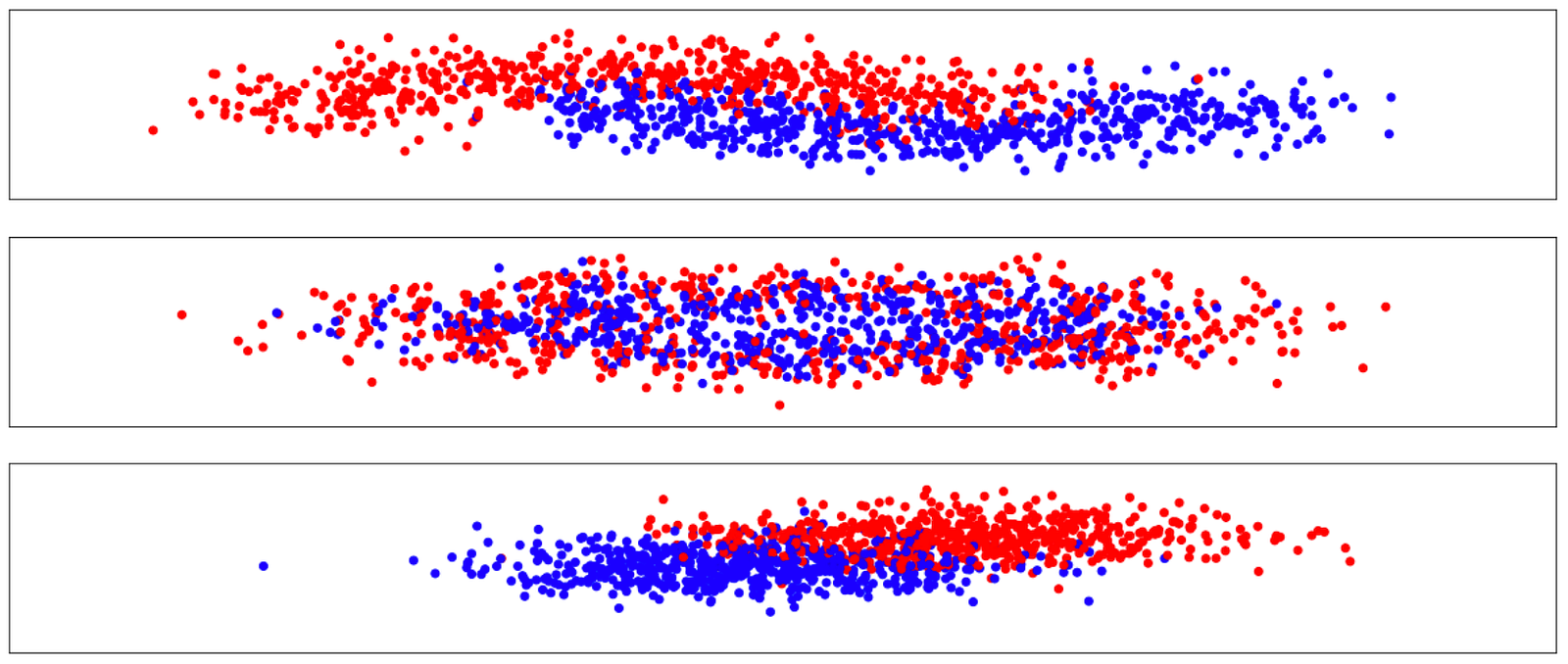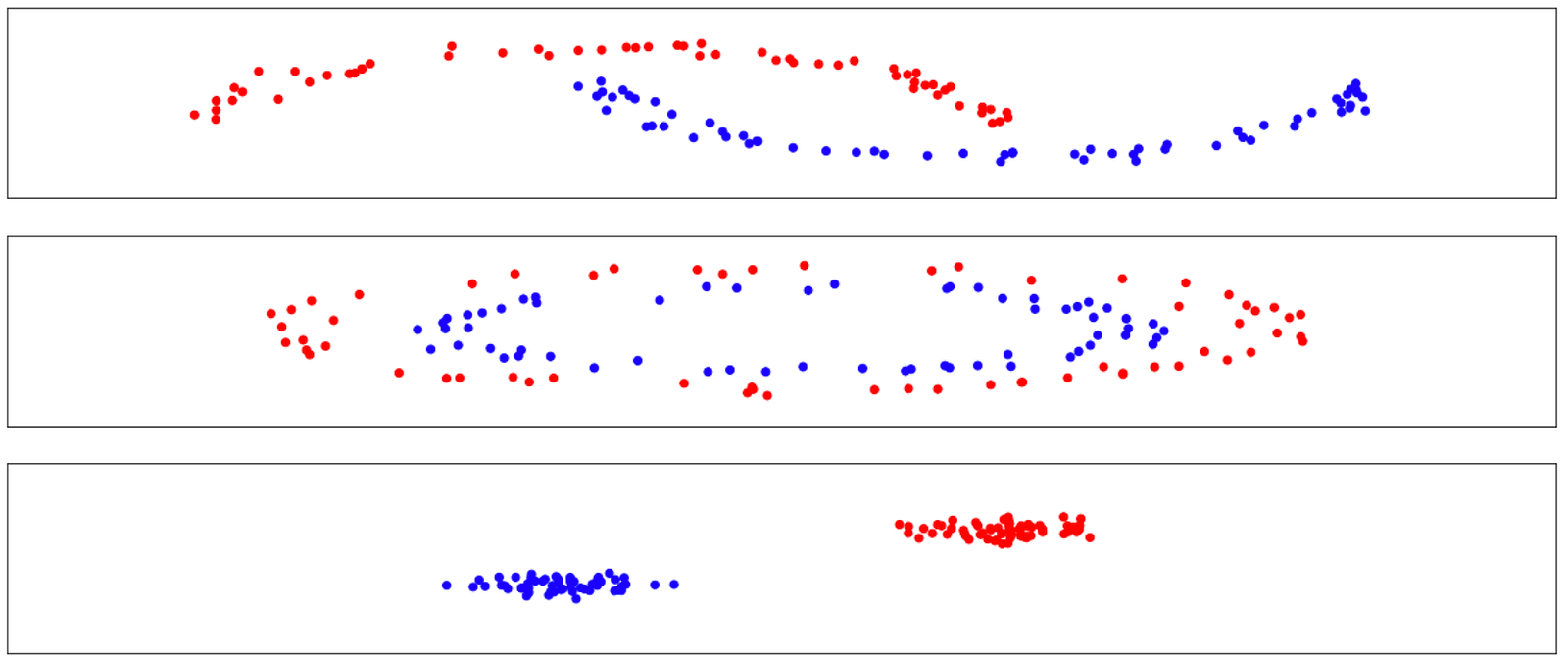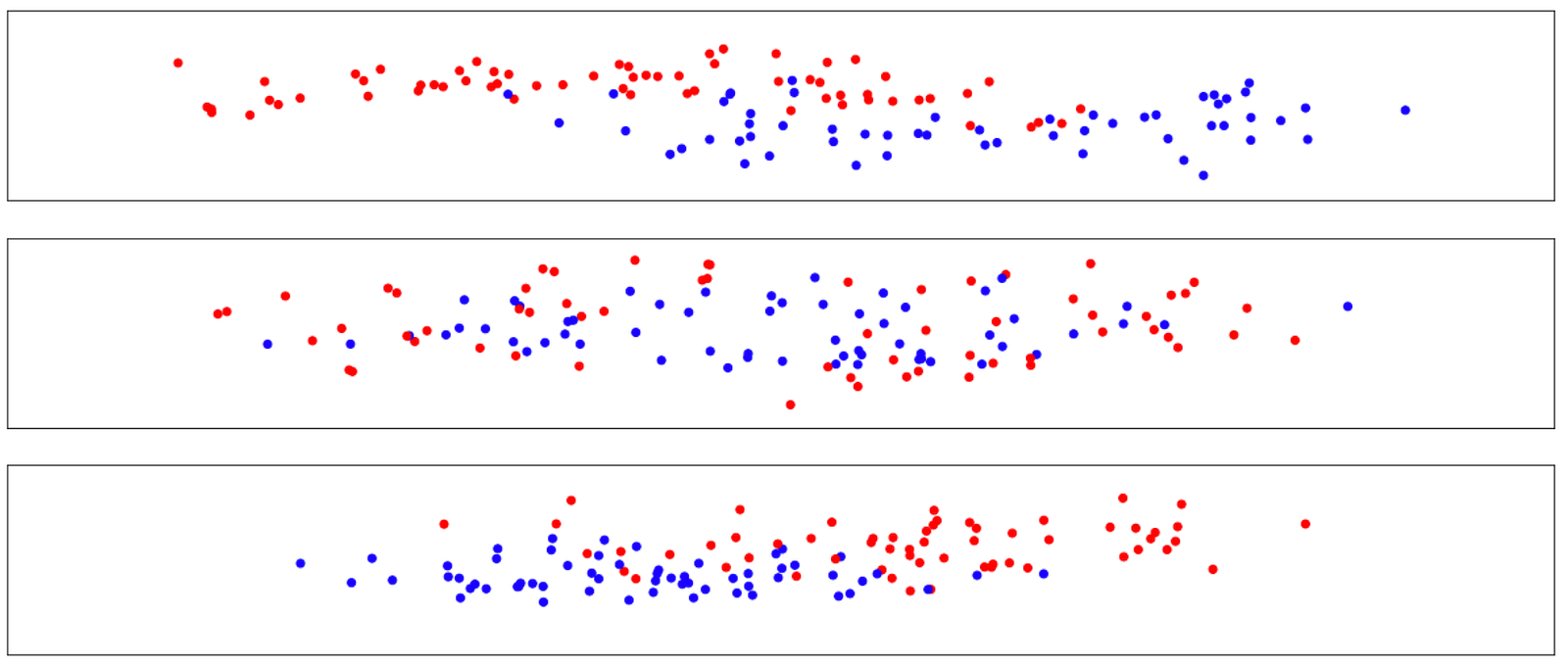Classification with OpenCV3 C++ (1/2)

OpenCV is an open source C++ library focusing on computer vision launched in 1999 by Intel research. It is written in C++ but bindings in Python and Matlab are available. The project has been supported by Willow Garage since 2008 and is under active development. OpenCV provides tools for many computer vision applications such as image/gesture recognition, motion tracking, mobile robotics… Computer vision is closely related to machine learning thus OpenCV has a module that implements many traditional algorithms. And more recently, OpenCV 3 added support for deep learning algorithms.

I decided to do some experiments quite close to the ones performed by Kolanovic  since I find that it allows to do some catch up on traditional ML while getting a little bit more familiar with the OpenCV C++ API. After finishing my experiments I decided to publish these results, they might be useful to anyone starting out with OpenCV C++. I personally prefer working with Opencv in C++, here is a post weighting the pros and cons of each interface available ( C++, Matlab or python).

Installing OpenCV3

I strongly recommend the macOS package manager Homebrew which makes life much easier and provides a clean, simple interface to build libraries, though some are not available (such as caffe). Some would argue that this gives the false impression that coding is simple, however, for beginners and people that are more scientists than programmers, and ideally would want to focus on the actual prototyping/experimenting phase than the actual setting up phase, Homebrew is perfect. For a C++11 installation with python binding and contrib modules the following command is needed:

`brew install opencv3 --c++11 --with-python3 --with-contrib`

The contrib modules are extensions to OpenCV built-in classes. There seems to be a problem with installing OpenCV3 with the python3 binding, this blog post deals with this issue (it seems to has been fixed in recent commits but just in case).

Data

I use dummy data generated by scikit-learn. Actually, everything except the training and prediction will be done with python, i.e. generating the data with sklearn, then training the OpenCV C++ models, perform some cross validation with the test data. Then back to python to finally display the results with seaboarn. This make preparation and analysis of the results quicker and thus I can spend more time on the actual machine learning part. Doing these with C++ would have been a bit more painful.

This gives some dummy data generators to work with:

`from sklearn.datasets import make_moons, make_circles, make_blobs`

The data are from 3 different generators, either with a small (400) or large (4000) sample size and with low (5%) or large (30%) standard deviation (in red class 0 and in blue class 1):large samples with low variance (top: data set 1, middle: data set 2 and bottom: data set 3)large samples with high variance (top: data set 1, middle: data set 2 and bottom: data set 3)small samples with low variance (top: data set 1, middle: data set 2 and bottom: data set 3)small samples with high variance (top: data set 1, middle: data set 2 and bottom: data set 3)

The data can then easily be saved to csv with pandas. You can find the notebook I used for visualisation here.

OpenCV containers

OpenCV has its own containers that are the input for their machine learning algorithms. Thus I only present the ones needed for this tutorial but the library has a rich collection of containers that are curated for computer vision applications.

The simplest container is the Mat data type that can be instantiated as follows:

`auto array = cv::Mat::ones(1, 20, CV_32F);`

Here a row vector with 20 columns is created with 1 as initial values for each element with a 32 bits precision. There are other functions allowing to initialise the matrix values:

`auto zer = cv::Mat::zeros(1, 20, CV_32F); // zero matrixauto id = cv::Mat::eye(20, 20, CV_32F); // identity matrix`

More generally, the matrix type is defined as follows for one channel matrices:

``CV_<bit_depth><type>``

The type can either be S for signed int, U for unsigned int and F for float. The bit depth can be 8, 16 32 or 64. OpenCV also allows for multi channel matrices that are designed to contain image pixels.

Inputs for machine learning algorithms in OpenCV are instances of the class TrainData. This class is quite useful because it has an internal train/test split logic thus the algorithm trained only the data only accesses the elements marked as trained and the remaining are discarded and used for testing. A set of sample data can be created as follows:

`auto X = cv::Mat::zeros(400,2,CV_32F); // dummy features matrixauto Y= cv::Mat::zeros(400,1,CV_32U); // dummy label matrixcv::Ptr<cv::ml::TrainData> data=cv::ml::TrainData::create(X,0,Y);`

where X is the instance cv::Mat with the observed features and Y a cv::Mat instance with the labels, both fed with the data generated by sklearn. The second parameter is 0 to signal that one observation is a row vector with the features as columns. If the value is 1 then one observation is a column vector. In order to set the train/test split ratio, the following member function is handy:

`data->setTrainTestSplitRatio(0.2,true);`

The first parameter defines the percentage set aside for testing and the functions performs a shuffle split is the second input value is true.

Binary classification

OpenCV C++ has a machine learning module wrapped in the cv::ml:: namespace. All the models implemented are derived class of the cv::ml::StatModel which declares training and prediction member functions for its instances. It is a pure virtual class and methods are accessed through pointers of the base class (dynamic polymorphism):

`cv::ml::Ptr<StatModel> model = cv::ml::StatModel::create();`

Thus the pointer of the base class StatModel allows to reference any of the model we want. The models can thus be trained nicely using the same member function:

`model->train(data);`

We can check at any moment whether a model was trained as follows:

`model->isTrained();`

When the model is trained, the generalisation error can be computed as follows:

`model->calcError(data, true, y);`

the first parameter takes as input the data set, the error is computed over the test set if the second parameter is true (generalisation error), otherwise the train set (training error). The last parameters outputs the labels predicted by the model for the set used. Instead of directly computing the error, it is also possible to compute prediction of a given set of features:

`model->predict(x_test);`

which returns a vector with the predicted classes.

Each model has its own parameter sets. So I go over the parameters I chose for the experiments. Some models have a grid search allowing to find the parameter set optimising the test classification accuracy. For the others, I just tweaked the parameters until I got to satisfying results.

Once the model is instantiated, its parameters needs to be set by the user, here are the parameters chosen for the following algorithms (I assume that these traditional algorithms are known by the reader):

`kNearest->setDefaultK(5);kNearest->setIsClassifier(true);`
• setDefaultK: sets the number of nearest neighbours.
• setIsClassifier: if false, the algorithm fitted is a regression (i.e. continuous output) if true it is a classifier.
`decisionTree->setMaxDepth(3000);decisionTree->setMinSampleCount(1);decisionTree->setUse1SERule(false);decisionTree->setUseSurrogates(false);decisionTree->setPriors(cv::Mat());decisionTree->setCVFolds(1);`
• setMaxDepth: sets the maximum depth of the tree.
• setMinSampleCount: sets the minimum number of observation in each leaf.
• setUse1SERule: is true algorithm performs a more aggressive pruning of the tree which reduces its variance.
• setUseSurrogates: only useful if there are missing input values.
• setPriors: skews penalty for miss-classification for certain classes.
• setCVFolds: sets the cross validation procedure order for pruning the tree.

I go into more details on decision trees in openCV C++ in an upcoming post.

random forest

`randomForest->setMaxCategories(2);randomForest->setMaxDepth(3000);randomForest->setMinSampleCount(1);randomForest->setTruncatePrunedTree(false);randomForest->setUse1SERule(false);randomForest->setUseSurrogates(false);randomForest->setPriors(cv::Mat());randomForest->setTermCriteria(criterRamdomF);randomForest->setCVFolds(1);`
• setTermCriteria: sets the convergence criteria by choosing when the algorithm stops (either when a given precision is reached CV_TERMCRIT_EPS, or when a number of iteration is reached CV_TERMCRIT_ITER). Then the value of each criteria must be set.
`auto criterRamdomF = cv::TermCriteria();criterRamdomF.type = CV_TERMCRIT_EPS + CV_TERMCRIT_ITER;criterRamdomF.epsilon = 1e-8;criterRamdomF.maxCount = 5000;`

boost

`boost->setBoostType(cv::ml::Boost::DISCRETE);boost->setWeakCount(100);boost->setMaxDepth(2000);boost->setUseSurrogates(false);boost->setPriors(cv::Mat());`
• setBoostType: selects the type of boosting algorithm used (options are AdaBoost or Logit).
• setWeakCount: sets the number of weak classifiers used.

linear SVM

`linearSvm->setC(100);linearSvm->setKernel(linearSvm->LINEAR);;linearSvm->setTermCriteria(criterSvm);linearSvm->setType(linearSvm->C_SVC);`
• setKernel: sets the kernel function.
• setC: sets the regularization hyperparameter.

rbf SVM

`rbfSvm->setC(1000);rbfSvm->setTermCriteria(criterRbf);rbfSvm->setCoef0(0.3);rbfSvm->setKernel(rbfSvm->RBF);rbfSvm->setGamma(0.9);rbfSvm->setType(rbfSvm->C_SVC);`
• setGamma: hyperparameter of the sigmoid kernel function.
• setCoef0: hyperparameter of the sigmoid kernel function.

sigmoid SVM

`sigmoidSvm->setC(1000);sigmoidSvm->setTermCriteria(criterSigmoid);sigmoidSvm->setCoef0(0.3);sigmoidSvm->setGamma(0.9);sigmoidSvm->setKernel(sigmoidSvm->SIGMOID);sigmoidSvm->setType(sigmoidSvm->C_SVC);`

I also visit further Support Vector Machines in Open CV C++in an upcoming post.